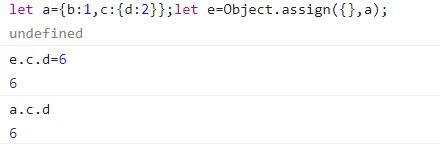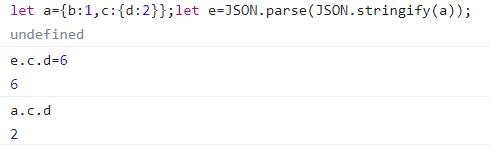# 浅谈前端js对象的深拷贝和浅拷贝

## 浅拷贝

``````{a: 1, b: 'xx', c: true}//number/string/boolean
``````

``````{a: 1, b: {c: 2}}
``````

”对一个对象进行浅拷贝，该对象下的基本类型属性会开辟新的空间，引用类型属性是还是指向同一块空间。”

• ##### Object.assign()
``````  let a = {b: 1,c: {d: 2}}
let e = Object.assign({}, a)

//1、改变a对象中的b，e对象的b不会变
a.b = 2
console.log(e.b)//1

//2、改变a对象中的c，e对象中的c也不会变
a.c = {d: 3}
console.log(e.c)//{d: 2}

//3、改变a对象第二层的对象d，e对象中的d也会变
a.c.d = 3
console.log(e.c.d)//3

//4、反过来，改变e对象中的d，a对象中的d也会变，如下图
e.c.d = 6
console.log(a.c.d)//6
``````## 深拷贝

• ##### JSON.parse(JSON.stringify())
``````  let a = {b: 1,c: {d: 2}}
let e = JSON.parse(JSON.stringify(a))

//1、改变a对象中的b，e对象的b不会变
a.b = 2
console.log(e.b)//1

//2、改变a对象中的c，e对象中的c也不会变
a.c = {d: 3}
console.log(e.c)//{d: 2}

//3、改变a对象第二层的对象d，e对象中的d也不会变
a.c.d = 3
console.log(e.c.d)//2

//4、反过来，改变e对象中的d，a对象中的d也不会变，如下图
e.c.d = 6
console.log(a.c.d)//2
``````734802480（已满）、 794324979

﻿